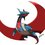# power proof

hey,guys,i'm practicing proofs .so, i'll be writing some proofs for the community to point out flaws, and many others to learn this are all about the golden ratios. often written as$$\phi \quad or\quad\varphi=\dfrac{1+\sqrt{5}}{2}$$ first is the power proof, i.e $\phi^n=F_{n}\phi+F_{n-1}$ where$$F_{n}$$ is the nth Fibonacci number, which is defined as $F_n=\begin{cases} 1,& n=1\\1,&n=2\\F_{n-1}+F_{n-2},&n\geq 3\end{cases}$ $the\quad proof$ we see that it is satisfied at n=1,2. we know that $\phi^2=\phi+1\longrightarrow \phi^n=\phi^{n-1}+\phi^{n-2}$ since $$\phi^{n-1}=F_{n-1}\phi+F_{n-2}, \phi^{n-2}=F_{n-2}\phi+F_{n-3}$$, $\begin{array} &\phi^n=\phi^{n-1}+\phi^{n-2}\\ \phi^n=F_{n-1}\phi+F_{n-2}+F_{n-2}\phi+F_{n-3}\\ \phi^n=(F_{n-1}+F_{n-2})\phi+(F_{n-2}+F_{n-3})\\ \phi^n=F_{n}\phi+F_{n-1} \end{array}$ since it satisfies all three at n,n-1,n-2.it must satisfy for all number. induction, hence proved.Note by Aareyan Manzoor
6 years, 5 months ago

This discussion board is a place to discuss our Daily Challenges and the math and science related to those challenges. Explanations are more than just a solution — they should explain the steps and thinking strategies that you used to obtain the solution. Comments should further the discussion of math and science.

When posting on Brilliant:

• Use the emojis to react to an explanation, whether you're congratulating a job well done , or just really confused .
• Ask specific questions about the challenge or the steps in somebody's explanation. Well-posed questions can add a lot to the discussion, but posting "I don't understand!" doesn't help anyone.
• Try to contribute something new to the discussion, whether it is an extension, generalization or other idea related to the challenge.

MarkdownAppears as
*italics* or _italics_ italics
**bold** or __bold__ bold
- bulleted- list
• bulleted
• list
1. numbered2. list
1. numbered
2. list
Note: you must add a full line of space before and after lists for them to show up correctly
paragraph 1paragraph 2

paragraph 1

paragraph 2

[example link](https://brilliant.org)example link
> This is a quote
This is a quote
    # I indented these lines
# 4 spaces, and now they show
# up as a code block.

print "hello world"
# I indented these lines
# 4 spaces, and now they show
# up as a code block.

print "hello world"
MathAppears as
Remember to wrap math in $$ ... $$ or $ ... $ to ensure proper formatting.
2 \times 3 $2 \times 3$
2^{34} $2^{34}$
a_{i-1} $a_{i-1}$
\frac{2}{3} $\frac{2}{3}$
\sqrt{2} $\sqrt{2}$
\sum_{i=1}^3 $\sum_{i=1}^3$
\sin \theta $\sin \theta$
\boxed{123} $\boxed{123}$

Sort by:

Hello Aareyan,

Your proof is perfectly okay and completely free of any flaw.

You might also want to check out the articles we have on induction and its variants.

Good luck to you in your quest of proving things!

- 6 years, 5 months ago

thanks,sir. i was worried about 2 cases.

- 6 years, 5 months ago

You are using a variant called 'Strong induction' that uses more statements in the inductive hypothesis.

And 'sir' sounds really formal. You can call me Mursalin if you want.

- 6 years, 5 months ago

thanks,sir. learned something new, and sir, i learned English through formal speaking.,so it is an habit.

- 6 years, 5 months ago